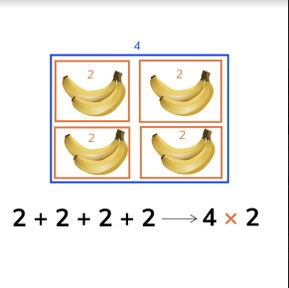Introduction to multiplication

# Introduction to multiplication

Students are introduced to multiplication by counting groups of objects.8,000 schools use Gynzy92,000 teachers use Gynzy1,600,000 students use Gynzy

## General

Students are introduced to multiplication. in the form of repeated addition problems, otherwise called 'times' problems. They are also introduced to the multiplication notation 'x'.

## Standards

CCSS.Math.Content.3.OA.A.1

## Learning objective

Students will be able to multiply numbers by counting in groups, in an introductory way.

## Introduction

Hand out number cards with the numbers 1 to 10 to the students. Have students make pairs. As a pair they must solve the addition problem that their number cards create.
Example: Jan and Ingrid have the numbers 9 and 4. The addition problem becomes 9 + 4. They solve this problem. Then they find a new partner and solve the next problem.

## Instruction

Ask students to count the objects. How many objects are there? Ask students which methods they can use to easily add the given numbers. Start by explaining what multiplication (or times) problems are problems in which you figure out how many times you have a group of something. Show that you have 2 strings with 4 beads. The times problem is 2 times 4. Next show the bananas. Show that each bunch of bananas has 2 bananas. If you create an addition problem it would become: 2 + 2 + 2 + 2. You can also say that you have 4 bunches of 2 bananas. Which is 4 times 2. Check that students are able to create times problems with the given groups of objects. Show students that a multiplication (or times) problem is created with the first number, which tells you how many times you have the group, and the second number, which shows how many objects are in the group. Check that students are able to solve the times problems. Then give instruction on how to write multiplication problems. Show students that the 'x' sign is used to show that you are writing a multiplication problem. Together with the students, match the multiplication problem to the addition problem by drawing a line between the two matching problems. Finally ask students to drag the correct math problem to the matching image.

Check that students understand the basics of multiplication by asking the following questions:
- What is a multiplication (or times) problem?
- How do you make a times problem out of 3 + 3 + 3?
What does 2 x 5 mean?

## Quiz

Students are given exercises in which they must match the given addition problem to its times problem, match an image to a problem, drag the addition and multiplication problem to matching categories, and determine the addition and multiplication problem shown by a number line.

## Closing

Discuss the importance of multiplying and times problems with students, reminding them that by learning how to do this, they are then able to solve large addition problems quickly. Ask students how they can turn a repeating addition problem into a times problem and how to solve it. Finally have students create their own times problems using objects in the classroom.
Example: Janey takes a handful of colored pencils. They make 4 groups of 3 colored pencils. Sam comes to solve this problem and creates 4 times 3, and solves to determine that there are 12 colored pencils. Then Sam gets another set of objects and makes a problem for Janey (or another student).

## Teaching tips

Be aware that students start to build familiarity with multiplication and times problems by visual representation of this concept. Have students use tangible materials (like blocks or markers) to create the given problems, or have them draw the groups of objects on a sheet of paper. If students have difficulty with this learning goal, they can also practice counting in jumps on a number line to represent the repeating addition. Also remind students that they are adding the same number over and over again, it does not change.

## Instruction materials

Number cards and blocks

### The online teaching platform for interactive whiteboards and displays in schools

• Save time building lessons

• Manage the classroom more efficiently

• Increase student engagement